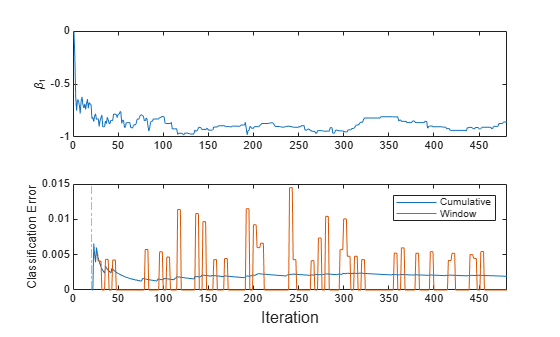# Implement Incremental Learning for Classification Using Succinct Workflow

This example shows how to use the succinct workflow to implement incremental learning for binary classification with prequential evaluation. Specifically, this example does the following:

1. Create a default incremental learning model for binary classification.

2. Simulate a data stream using a for loop, which feeds small chunks of observations to the incremental learning algorithm.

3. For each chunk, use updateMetricsAndFit to measure the model performance given the incoming data, and then fit the model to that data.

Although this example treats the application as a binary classification problem, you can implement multiclass incremental learning using an object for a multiclass problem by following this same workflow.

### Create Default Model Object

Create a default incremental learning model for binary classification.

Mdl = incrementalClassificationLinear()
Mdl = incrementalClassificationLinear IsWarm: 0 Metrics: [1x2 table] ClassNames: [1x0 double] ScoreTransform: 'none' Beta: [0x1 double] Bias: 0 Learner: 'svm' Properties, Methods 

Mdl is an incrementalClassificationLinear model object. All its properties are read-only.

Mdl must be fit to data before you can use it to perform any other operations.

Load the human activity data set. Randomly shuffle the data.

load humanactivity n = numel(actid); rng(1) % For reproducibility idx = randsample(n,n); X = feat(idx,:); Y = actid(idx);

For details on the data set, enter Description at the command line.

Responses can be one of five classes: Sitting, Standing, Walking, Running, or Dancing. Dichotomize the response by identifying whether the subject is moving (actid > 2).

Y = Y > 2;

### Implement Incremental Learning

Use the succinct workflow to update model performance metrics and fit the incremental model to the training data by calling the updateMetricsAndFit function. At each iteration:

• Process 50 observations to simulate a data stream.

• Overwrite the previous incremental model with a new one fitted to the incoming observations.

• Store the cumulative metrics, the window metrics, and the first coefficient ${\beta }_{1}$ to see how they evolve during incremental learning.

% Preallocation numObsPerChunk = 50; nchunk = floor(n/numObsPerChunk); ce = array2table(zeros(nchunk,2),'VariableNames',["Cumulative" "Window"]); beta1 = zeros(nchunk,1); % Incremental fitting for j = 1:nchunk ibegin = min(n,numObsPerChunk*(j-1) + 1); iend = min(n,numObsPerChunk*j); idx = ibegin:iend; Mdl = updateMetricsAndFit(Mdl,X(idx,:),Y(idx)); ce{j,:} = Mdl.Metrics{"ClassificationError",:}; beta1(j + 1) = Mdl.Beta(1); end

Mdl is an incrementalClassificationLinear model object trained on all the data in the stream. During incremental learning and after the model is warmed up, updateMetricsAndFit checks the performance of the model on the incoming observations, and then fits the model to those observations.

### Inspect Model Evolution

To see how the performance metrics and ${\beta }_{1}$ evolve during training, plot them on separate tiles.

t = tiledlayout(2,1); nexttile plot(beta1) ylabel('\beta_1') xlim([0 nchunk]) nexttile h = plot(ce.Variables); xlim([0 nchunk]) ylabel('Classification Error') xline(Mdl.MetricsWarmupPeriod/numObsPerChunk,'g-.') legend(h,ce.Properties.VariableNames) xlabel(t,'Iteration')The plot suggests that updateMetricsAndFit does the following:

• Fit ${\beta }_{1}$ during all incremental learning iterations.

• Compute the performance metrics after the metrics warm-up period only.

• Compute the cumulative metrics during each iteration.

• Compute the window metrics after processing 200 observations (4 iterations).